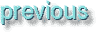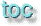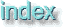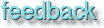Whole document tree

Whole document tree

GUM v.1.0.0# Index

Numerics
4-color [O]
8-bit [O]
A
active layer [O]
airbrush [O], [O]
brush stroke [O]
pressure [O]
alien map [O]
align
horizontal [O]
vertical [O]
align visible layers [O]
allow window resizing [O], [O]
alpha
channel [O]
channel what is [O]
decomposing [O]
holes [O]
selection edit [O]
selection store [O]
to selection [O]
web image [O]
alpha to selection [O]
AM screening [O]
animation gif [O]
animation optimize [O]
animation playback [O]
animframe [O]
basic concept [O]
beyond basics [O]
concept basic [O]
controls [O]
convert [O]
duplicate [O]
exchange [O]
flatten [O]
gallery [O]
how to [O]
layerdel [O]
make frame [O]
move path [O]
navigate frames [O]
preview [O], [O]
source select [O]
to image [O]
tutorial [O]
undo [O]
antialiasing [O]
apply canvas [O]
apply lens [O]
Authors xxxix
Karin Kylander xxxix
Olof S Kylander xxxix
autocrop [O]
auto-stretch hsv [O]
B
background [O]
color [O]
balance [O]
bbs [O]
behind mode [O]
bezier [O], [O]
control points move [O]
sharp corners [O]
bilinear [O]
black level calibration [O]
blackness [O]
blend [O]
blend tool [O], [O]
bilinear [O]
color options [O]
fill [O]
linear [O]
offset [O]
sawtooth [O]
shapeburst [O]
square [O]
symmetric asymmetric [O]
triangular [O]
blinds [O]
blur [O], [O]
gaussian [O]
iir [O]
motion [O]
rle [O]
variable [O]
blurring [O]
bmp [O]
border [O], [O], [O]
brightness [O]
brush
dialog [O]
directory [O]
make [O]
name [O]
opacity [O]
pop up [O]
spacing [O]
stroke [O]
brush selection [O], [O]
mode [O]
opacity [O]
spacing [O]
bucket fill [O], [O]
fill opacity [O]
fill threshold [O]
mode [O]
pattern fill [O]
sample merged [O]
bump map [O]
bzip [O]
C
calibration [O]
black level [O]
color [O]
gamma [O]
white level [O]
carve it [O]
cel [O]
central reflection [O]
Changelog xliii
channel [O]
alpha [O]
alpha edit [O]
alpha selection [O]
alpha what is [O]
layers [O]
rgb [O]
save to [O]
channel ops [O]
checkerboard [O]
chrome it [O]
clear [O]
clone [O]
retouch [O]
close [O]
cml explorer [O]
cms [O]
Caldera [O]
Mentalix [O]
cmy [O]
cmyk [O], [O]
decomposing [O]
code [O]
color [O], [O], [O]
balance [O]
brightness-contrast [O]
calibration [O]
calibration cms [O]
calibration Frozenriver [O]
calibration plain [O]
calibration poor man [O]
calibration rgb & cmyk [O]
cmyk decomposing [O]
colorify [O]
compose [O]
contrast auto stretch [O]
curves [O]
curves workflow [O]
decompose [O]
desaturate i.e "grayscale" [O]
dialog [O]
exchange [O]
filter pack [O]
gamut [O]
highlights [O]
hot colors [O]
hsv decomposing [O]
hue [O]
hue hints [O]
invert [O]
levels [O]
levels examples [O]
levels rgb or alpha [O]
midtone [O]
mode [O]
normalize [O]
posterize [O]
rotate [O]
saturation [O]
selection [O]
selection, modes [O]
threshold [O]
color calibration [O]
color calibration cms [O]
color calibration Frozenriver [O]
color calibration plain [O]
color calibration poor man [O]
color calibration rgb & cmyk [O]
color dialog [O]
color exchange [O]
color gamut [O]
color info [O]
color management [O]
cms [O]
gamut [O]
profile [O]
rgb & cmyk [O]
color map rotation [O]
color mode [O]
color models [O]
cmy [O]
cmyk [O]
complementary colors [O]
grayscale [O]
hsv [O]
indexed [O]
indexed gif [O]
inverted colors [O]
munsell [O]
natural color system [O]
ncs [O]
Pantone [O]
rgb [O]
spot color [O]
subtractive [O]
Truematch [O]
color picker [O]
color info [O]
sample merged [O]
color proof [O]
color select [O]
colorify [O]
coloring [O]
combine
depth merge [O]
file [O]
fuse [O]
comparing different modes [O]
compile [O]
code [O]
configure [O]
flag see flag
gcc [O]
how to [O]
include files [O]
libraries [O]
makefile [O]
multiple source files [O]
programs [O]
variables [O]
complementary colors [O]
compose [O]
convolver [O]
blurring [O]
sharpen [O]
configure [O], [O]
configure flags [O]
conical anamorphose [O]
contrast [O]
contrast auto stretch [O]
Contributions xli
Gimp contributions xli
GUM contributions xlii
convert
grayscale [O]
indexed [O]
rgb [O]
convolution matrix [O]
coordinate map [O]
copy [O]
named [O]
visible [O]
correct color [O]
creating image objects [O]
crop [O]
move [O]
nudge [O]
resize [O]
selection [O]
snap to guides [O]
crypt [O]
cubism [O]
curtain [O]
curves [O]
workflow [O]
cut [O]
named [O]
D
darken mode [O]
db browser [O]
decompose [O]
decompress [O]
decrypt [O]
deinterlace [O]
delete layer [O]
desaturate [O]
despeckle [O]
destripe [O]
difference mode [O], [O]
diffraction patterns [O]
digital signature [O]
displace [O]
black [O]
calculations [O]
description [O]
examples [O]
smear [O]
tips & tricks [O]
wrap [O]
dissolve mode [O]
dithering [O]
dots [O]
dpi [O], [O]
draw [O]
duplicate [O]
duplicate layers [O]
E
edge [O]
edge detect [O]
emboss [O]
encoding [O]
encrypt [O]
engrave [O]
eps [O], [O], [O]
resolution [O]
equalize [O]
eraser [O]
eye [O]
Ezdrive [O]
F
family [O]
faxG3 [O]
feather [O], [O]
FF [O]
fig [O]
figures [O]
file formats [O], [O]
bmp [O]
bzip [O]
cel [O]
eps [O], [O], [O]
faxG3 [O]
fits [O]
fli [O]
gbr [O], [O]
gicon [O], [O]
gif [O], [O]
gzip [O]
hrz [O]
jpeg [O], [O]
mpeg [O]
pat [O], [O]
pcx [O]
pdf [O], [O]
pix [O]
png [O], [O]
pnm [O], [O]
postscript [O]
ps [O], [O]
psd [O]
sgi [O]
snp [O]
sunras [O], [O]
tga [O], [O]
tiff [O], [O], [O]
url [O]
xcf [O], [O]
xpm [O], [O]
xwd [O]
file transfer [O]
filesystem format [O]
fill [O]
opacity [O]
threshold [O]
film [O]
how to [O]
filter all layers [O]
filter factory [O]
filters
alien map [O]
all layers [O]
animation optimize [O]
animation playback [O]
apply lens [O]
artistic [O]
blinds [O]
blur [O], [O]
blur gaussian [O]
blur iir [O]
blur motion [O]
blur rle [O]
blur variable [O]
bump map [O]
bump map usage [O]
canvas [O]
central reflection [O]
checkerboard [O]
cml explorer [O]
color exchange [O]
color filter pack [O]
color map rotation [O]
colorify [O]
conical anamorphose [O]
convolution matrix [O]
coordinate map [O]
crypt [O]
cubism [O]
curtain [O]
deinterlace [O]
depth merge [O]
despeckle [O]
destripe [O]
diffraction patterns [O]
digital signature [O]
displace [O]
displace see displace
edge [O]
edge detect [O]
emboss [O]
engrave [O]
figures [O]
film [O]
filter factory [O]
filter pack [O]
filter pack color [O]
flame [O]
flarefx [O]
fractal trace [O]
fuse [O]
gfig [O]
gflare [O]
gif animation [O]
glass tile [O]
grid [O]
hide file [O]
hot (colors) [O]
ifs compose [O]
illusion [O]
iwrap [O]
laplace [O]
lic [O]
lic how to [O]
light effect [O]
make seamless [O]
map object [O]
mathmap [O]
max rgb [O]
maze [O]
mosaic [O]
mosaic tip [O]
motion blur [O]
nl [O]
oilify [O]
paper tile [O]
pinch [O]
pixelize [O]
plasma [O]
polar coords [O]
qbist [O]
quantize [O]
randomize [O]
refract [O]
ripple [O]
scatter hsv [O]
semi flatten [O]
sharpen [O]
shift [O]
sinus [O]
small tile [O]
smooth palette [O]
sobel [O]
solid noise [O]
sparkle [O]
stegano [O]
stereogram [O]
super nova [O]
texture [O]
tile [O]
twist [O], [O]
universal [O]
user [O]
value propagate [O]
van gogh [O]
van gogh how to [O]
variable blur [O]
video [O]
warp [O]
waves [O]
whirl [O]
fits [O]
flag [O]
cflags [O]
configure flags [O]
-I [O]
--includedir [O]
-L [O]
ldflags [O]
--libdir [O]
-o [O]
flame [O]
flare
flarefx [O]
gflare [O]
flarefx [O]
flatten [O], [O]
flatten image [O]
flatten semi [O]
fli [O]
flip [O], [O]
float [O]
floating selection
tips [O]
floating selection
what is [O]
FM screening [O]
font [O], [O]
copy [O]
encoding [O]
family [O]
file [O]
foundry [O]
install [O]
management [O]
origin [O]
path [O]
scalable [O]
slant [O]
typ1inst [O]
type 1 [O]
weight [O]
width [O]
fonts how they work [O]
foreground color [O]
foundry [O], [O]
fractal trace [O]
Frozenriver xl
ftp [O]
fuse [O]
G
gallery [O]
gamma calibration [O]
gaussian blur [O]
gbr [O], [O]
gcc [O]
gfig [O]
brush [O]
curves [O]
directory [O]
example [O]
lines [O]
options [O]
paint [O]
preview [O]
select [O]
settings [O]
user interface [O]
gflare [O]
directory [O]
ghostscript [O], [O]
gicon [O], [O]
gif [O], [O]
animation [O], [O]
animation loop [O]
comment [O]
interlaced [O]
save [O]
transparent background color [O]
Gimp
authors [O]
features [O]
future [O]
history 0.54 [O]
history 0.60 [O]
history 0.99 [O]
history 1.0 [O]
gimprc [O]
glass tile [O]
directory [O]
editor [O]
map [O], [O]
blend [O]
coloring [O]
endpoint color [O]
flip [O]
points [O]
pov-ray [O]
replicate [O]
save [O]
section [O]
segments [O]
segments split [O]
grayscale [O], [O]
convert [O]
grayscale levels [O]
grid [O]
grow [O]
guash [O], [O]
guides [O], [O]
snap [O]
toggle [O]
Gum
conventions xlvii
usage xlv
gunzip [O]
GYUM xl
gzip [O], [O], [O]
H
halftone [O]
cell [O]
dot [O]
matrix [O]
highlights [O]
histogram [O]
holes [O]
hot [O]
how to compile [O]
how to lic [O]
how to make a make file [O]
how to write script-fu [O]
hrz [O]
hsv [O], [O]
auto stretch [O]
decomposing [O]
hue [O]
saturation [O]
scatter [O]
value [O]
hue [O], [O], [O], [O]
hints [O]
hue mode [O]
I
ifs [O]
example [O]
options [O]
settings [O]
usage [O]
ifs compose see ifs
iir [O]
illusion [O]
image
auto correction [O], [O]
auto-stretch hsv [O]
background [O]
brightness [O]
channel ops [O]
color [O]
color balance [O]
compose [O]
contrast [O]
contrast auto stretch [O]
convert [O]
correct color [O]
curves [O]
decompose [O]
desaturate [O]
duplicate [O]
equalize [O]
flatten [O]
guash [O]
histogram [O]
hsv [O]
indexed [O]
invert [O]
line art [O]
mail [O]
new [O]
normalize [O]
objects create [O]
open [O]
open guash [O]
open postscript [O]
open type [O]
open type automatic [O]
posterize [O]
print [O]
resize [O]
rgb [O]
rotate [O]
saturation [O]
save [O]
save by extension [O]
save flatten [O]
save formats supported [O]
scale [O]
transparent [O]
image size [O]
image table [O]
import layers [O]
include files [O]
indexed [O], [O]
convert [O]
convert options [O]
custom palette [O]
dithering [O]
options [O]
posterize [O]
quantize [O]
install
.gimp [O]
binary [O]
bzip [O]
configure [O]
directory brushes [O]
directory gfig [O]
directory gflare [O]
directory palettes [O]
directory pattern [O]
directory plug-ins [O]
directory script-fu [O]
extra data [O]
font [O]
fonts [O]
fonts by hand [O]
gimp-1.0.X.tar.gz [O]
gimprc [O]
GNU ghostscript [O]
gzip [O]
libraries [O]
libgtk [O]
make [O]
personal files [O]
sane [O]
script-fu [O]
shared data [O]
source [O]
wget [O]
xv [O]
intelligent scissors [O]
interpolation [O], [O]
invert [O], [O]
inverted colors [O]
Iomega [O]
iwrap [O]
J
jaz [O]
jpeg [O], [O]
compression [O]
K
Karin Kylander xxxix
Key [O]
L
laplace [O]
layer
anchor [O]
layers [O]
active [O]
align visible [O]
alpha to selection [O]
channel [O]
copy visible [O]
delete [O]
dialog [O]
duplicate [O]
eye [O]
filter [O]
flatten [O]
import [O], [O]
introduction [O]
layerdel [O]
lower [O]
merge visible [O]
move [O]
naming [O]
new [O]
raise [O]
resize [O]
rotate [O]
scale [O]
symbols explanation [O]
thumbnail icon [O]
Legals i-- iii
Printing ii
levels [O], [O]
examples [O]
lic [O]
light effect [O]
lighten mode [O]
line art [O], [O]
linear [O]
lower layers [O]
lpi [O], [O]
lpi table [O]
M
magnifying [O]
allow window resizing [O]
new view [O]
shrink warp [O]
mail [O]
make [O], [O]
make seamless [O]
makefile [O]
example [O]
how to [O]
map object [O]
mathmap [O]
max rgb [O]
maze [O]
merge visible layers [O]
midtone [O]
mode [O], [O]
behind [O]
color [O], [O]
comparing different modes [O]
darken [O]
darken only [O]
difference [O], [O]
dissolve [O]
explanation between color, hue [O]
explanation between multiply, darken [O]
explanation between screen, addition, lighten [O]
few colors screen, addition, lighten [O]
hue [O], [O]
lighten [O]
lighten only [O]
multiply [O], [O], [O]
normal [O]
overlay [O], [O]
saturation [O], [O]
screen [O], [O]
subtraction [O]
value [O]
modem [O]
modes
what is [O]
mosaic [O]
mosaic tip [O]
Motion [O]
move [O], [O], [O]
float [O]
floating selection [O]
hints [O]
hole image [O]
layers [O]
nudge [O]
object floating selection [O]
path [O]
selections [O]
mpeg [O]
multiply mode [O], [O]
munsell [O]
N
name [O]
naming layers [O]
natural color system [O]
new layer [O]
new view [O], [O]
nl filter [O]
normal mode [O]
normalize [O]
nudge [O], [O]
O
offset [O]
move [O]
tile [O]
tip [O]
oil paint [O]
oilify [O]
Olof S Kylander xxxix
opacity [O], [O]
overlay mode [O], [O]
P
paintbrush [O]
palette [O], [O], [O]
create from image [O]
delete [O]
dialog [O]
directory [O]
edit [O]
from image [O]
hints [O]
new [O]
save [O]
Pantone [O], [O]
paper tile [O]
paste [O]
into [O]
named [O]
pat [O], [O]
pattern
directory [O]
make [O]
pattern fill [O]
pcx [O]
pdf [O]
pencil [O]
perspective [O], [O]
pinch [O]
pix [O]
pixelize [O]
plasma [O]
plug-in
compile programs [O]
plug-ins [O], [O]
categories [O]
compile [O]
configure [O]
directory [O]
filters [O]
gcc [O]
make [O]
what is [O]
png [O], [O]
pnm [O], [O]
pop up [O]
postscript [O]
power goo [O]
ppi [O], [O], [O]
preference [O]
directories [O]
display [O]
display interpolation [O]
environment [O]
interface [O]
suggestions [O]
swap [O]
preparing prepress [O]
prepress [O]
AM screening [O]
bbs [O]
Caldera [O]
calibration [O]
calibration black level [O]
calibration gamma [O]
calibration white level [O]
color [O]
color calibration [O]
color calibration cms [O]
color calibration Frozenriver [O]
color calibration plain [O]
color calibration poor man [O]
color calibration rgb & cmyk [O]
color management [O]
color proof [O]
dots [O]
dpi [O], [O]
email [O]
eps [O]
file formats [O]
file transfer [O]
filesystem format [O]
FM screening [O]
ftp [O]
halftone [O]
halftone cell [O]
halftone dot [O]
halftone matrix [O]
image size [O]
image table [O]
internet [O]
interpolation [O]
lpi [O], [O]
lpi table [O], [O]
Mentalix [O]
modem [O]
Pantone [O]
ppi [O], [O], [O]
preparing [O]
printer table [O]
printing [O]
removable drives [O]
resolution [O], [O]
resolution grid [O]
sane [O]
scan resolution [O]
scanning [O]
scanning Caldera [O]
scanning Mentalix [O]
scanning resolution [O]
scanning sane [O]
scanning XVscan [O]
screen frequencies [O]
screening matrix [O]
spot color [O]
stochastic screening [O]
tiff [O]
XVscan [O]
pressure [O]
primary [O]
print [O]
ghostscript [O]
settings [O]
supported printers [O]
printer table [O]
printing [O]
profile [O]
ps [O], [O]
resolution [O]
psd [O]
Q
qbist [O]
quantize [O]
quit [O]
R
raise layer [O]
randomize [O]
redo [O]
refract [O]
removable drives [O]
replicate [O]
resize [O], [O]
resize layer [O]
resolution [O], [O]
grid [O]
retouch [O]
rgb [O], [O], [O], [O]
channels [O]
composing [O]
convert [O]
decomposing [O]
invert [O]
levels [O]
max [O]
ripple [O]
rle [O]
rotate [O], [O]
rulers [O]
S
sample merged [O], [O], [O]
sane [O], [O]
saturation [O], [O], [O]
mode [O]
saturation mode [O]
save [O], [O]
native file format [O]
palette [O]
to channel [O]
sawtooth [O]
scale [O], [O]
scale layer [O]
scanning resolution [O], [O]
scatter hsv [O]
screen mode [O]
screen frequency [O]
screen shot [O]
screening matrix [O]
script-fu [O]
carve-it [O]
chrome-it [O]
directory [O]
don't and do:s [O]
image dependent [O]
install [O]
kinds of [O]
perspective [O]
stand alone [O]
what is [O]
script-fu how to write [O]
section [O]
select all [O]
select by color [O]
select by color modes [O]
select by color options [O]
select non [O]
selection [O], [O]
all [O]
alpha [O]
alpha edit [O]
alpha store [O]
antialiasing [O]
bezier [O]
bezier control points [O]
bezier control points move [O]
bezier sharp corners [O]
border [O]
by color [O]
by color options [O]
by color, modes [O]
color [O]
control [O]
difference [O]
ellipse [O]
feather [O], [O]
float [O]
floating move [O]
floating tips [O]
floating what is [O]
free-hand [O]
fuzzy [O]
gfig [O]
grow [O]
guide [O]
intelligent scissors [O]
intersection [O]
invert [O]
move [O]
non [O]
options [O]
rectangular [O]
sharpen [O]
shrink [O]
toggle [O], [O]
union [O]
semi flatten [O]
sgi [O]
shapeburst [O]
sharpen [O], [O], [O]
shearing [O]
shift [O]
short cuts [O]-- [O]
shrink [O]
shrink warp [O]
shrink wrap [O]
sinus [O]
slant [O], [O]
small tile [O]
smooth palette [O]
smoothing [O]
snap to guides [O]
anchor layer [O]
snp [O]
sobel [O]
solid noise [O]
source multiple [O]
source unpack [O]
spacing [O]
sparkle [O]
spot color [O], [O]
square [O]
stegano [O]
stereogram [O]
stochastic screening [O]
stroke [O]
brush selection [O]
subtraction mode [O]
sunras [O], [O]
super nova [O]
support
commercial xl
symbols [O]
symmetric asymmetric [O]
SyQuest [O]
T
tar [O]
text [O]
text tool [O]
blackness [O]
border [O], [O]
c [O]
foundry [O]
italic [O]
m [O]
p [O]
regular [O]
roman [O]
slant [O]
spacing [O]
weight [O]
width [O]
texture explorer [O]
textures [O], [O], [O], [O], [O]
tga [O], [O]
threshold [O], [O]
alpha [O]
thumbnail icon [O]
tiff [O], [O], [O]
compression [O]
fill order [O]
mac [O]
pc [O]
tile [O]
offset [O]
tile small [O]
tip of the day [O]
TODO xliv
toggle [O], [O], [O]
marching ants [O]
transform [O]
autocrop [O]
options [O]
perspective [O]
rotate [O]
scale [O]
shearing [O]
smoothing [O]
zealous crop [O]
triangular [O]
truecolor [O]
Truematch [O]
twist [O], [O]
U
undo [O], [O]
universal filter [O]
unpack [O]
url [O]
user filter [O]
V
value [O]
value mode [O]
value propagate [O]
van gogh [O]
variables [O]
video [O]
W
warp [O]
water colors [O]
watercolor [O]
waterselect [O]
waves [O]
weight [O]
wget [O]
what is modes [O]
whirl [O]
white level calibration [O]
width [O], [O]
weight [O]
window info [O]
X
xcf [O], [O]
xpm [O], [O]
XVscan [O]
xwd [O]
Z
zealous crop [O]
zip [O]
zoom [O], [O]
allow window resizing [O], [O]

Generated by fmtoweb (v. 2.9c) written by Peter G. Martin <peterm@zeta.org.au> Last modified: 20 May 1998